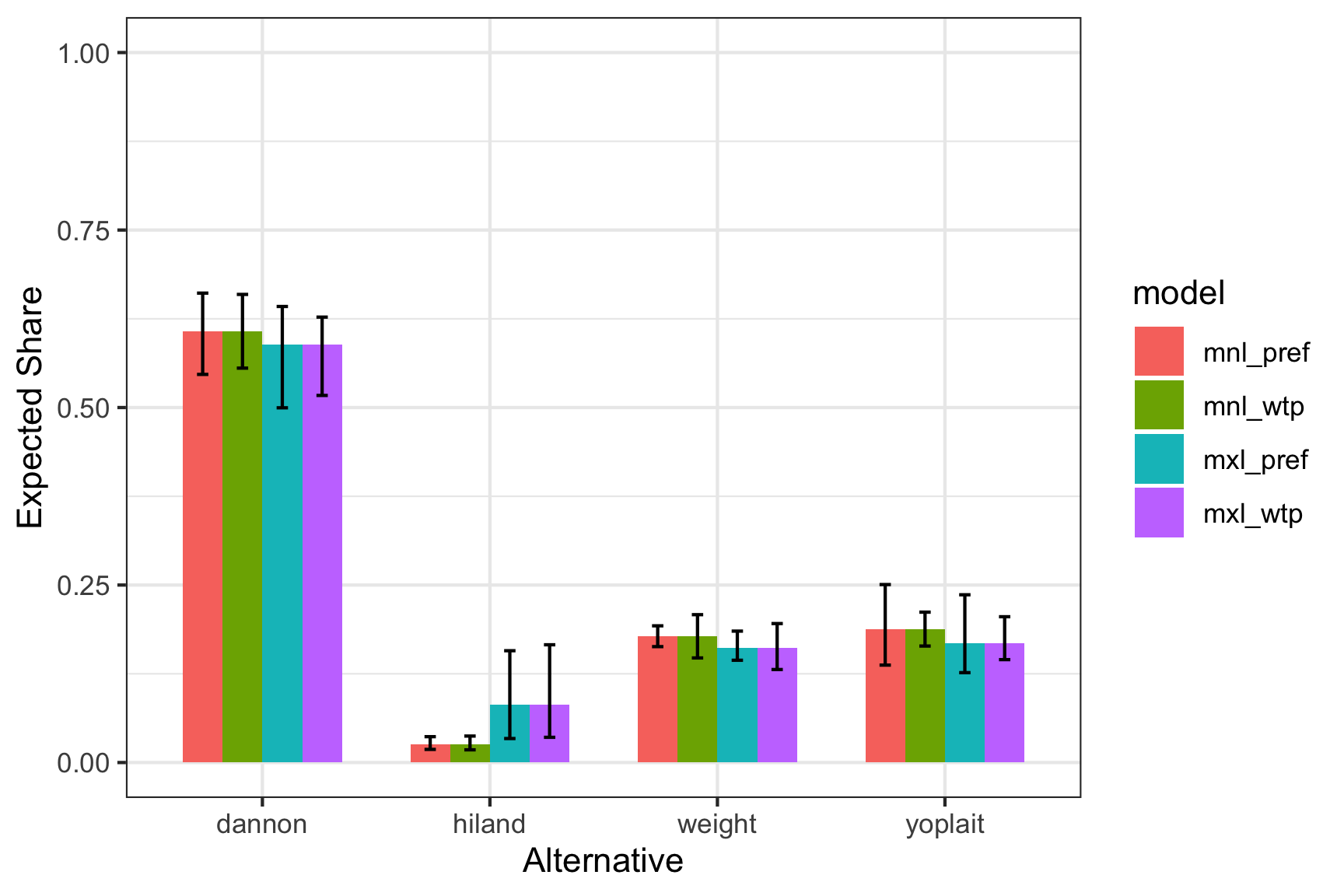# Simulating Shares from Estimated Models

Once a model has been estimated, it can be used to simulate the predicted shares for a set of alternatives. This vignette demonstrates examples of how to so using the simulateShares() function along with the results of an estimated model.

# The data

This example uses the yogurt data set from Jain et al. (1994). The data set contains 2,412 choice observations from a series of yogurt purchases by a panel of 100 households in Springfield, Missouri, over a roughly two-year period. The data were collected by optical scanners and contain information about the price, brand, and a “feature” variable, which identifies whether a newspaper advertisement was shown to the customer. There are four brands of yogurt: Yoplait, Dannon, Weight Watchers, and Hiland, with market shares of 34%, 40%, 23% and 3%, respectively.

# Simulating shares from estimated models

To simulate shares, you first need to create a set of alternatives where each row is an alternative and each column an attribute. In this example, I’ll just use one of the choice observations from the yogurt dataset:

alts <- subset(yogurt, obsID == 42,
select = c('feat', 'price', 'hiland', 'weight', 'yoplait'))
row.names(alts) <- c('dannon', 'hiland', 'weight', 'yoplait')
alts
#>         feat price hiland weight yoplait
#> dannon     0   6.3      0      0       0
#> hiland     1   6.1      1      0       0
#> weight     0   7.9      0      1       0
#> yoplait    0  11.5      0      0       1

Let’s say we have estimated a preference space MNL model called mnl_pref. We can use the simulateShares() function with the mnl_pref model to predict the shares for our alts set of alternatives:

sim_mnl_pref <- simulateShares(mnl_pref, alts, alpha = 0.025)
sim_mnl_pref
#>              share_mean  share_low share_high
#> Alt: dannon  0.60766441 0.54666931 0.66116286
#> Alt: hiland  0.02601869 0.01849724 0.03641177
#> Alt: weight  0.17802424 0.16324761 0.19255476
#> Alt: yoplait 0.18829266 0.13721551 0.25067896

The results show the expected shares for each alternative. The low and high values show a 95% confidence interval, estimated using simulation. You can change the CI level by setting alpha to a different value (e.g. a 90% CI is obtained with alpha = 0.05).

You can also use WTP space models to simulate shares, but you must provide the additional priceName argument to the simulateShares() function. For example, here are the results from an equivalent model but in the WTP space:

sim_mnl_wtp <- simulateShares(mnl_wtp, alts, priceName = 'price')
#> NOTE: Using results from run 6 of 10 multistart runs
#> (the run with the largest log-likelihood value)
sim_mnl_wtp
#>              share_mean  share_low share_high
#> Alt: dannon  0.60767403 0.55551255  0.6593344
#> Alt: hiland  0.02601652 0.01790205  0.0372950
#> Alt: weight  0.17802391 0.14731657  0.2082626
#> Alt: yoplait 0.18828554 0.16406714  0.2118064

Since these two models are equivalent except in different spaces, the simulation results should be the same.

Simulations also work with mixed logit models, and they account for how heterogeneity is modeled by simulating draws from the population estimates of the estimated model:

sim_mxl_pref <- simulateShares(mxl_pref, alts)
sim_mxl_pref
#>              share_mean  share_low share_high
#> Alt: dannon  0.58853780 0.49963606  0.6423508
#> Alt: hiland  0.08172329 0.03379753  0.1574826
#> Alt: weight  0.16135077 0.14406118  0.1850985
#> Alt: yoplait 0.16838815 0.12658930  0.2362842

Likewise, mixed logit WTP space models can also be used to simulate shares:

sim_mxl_wtp <- simulateShares(mxl_wtp, alts, priceName = 'price')
sim_mxl_wtp
#>              share_mean  share_low share_high
#> Alt: dannon  0.58876362 0.51704108  0.6273589
#> Alt: hiland  0.08138807 0.03551802  0.1658617
#> Alt: weight  0.16137402 0.13099899  0.1957457
#> Alt: yoplait 0.16847429 0.14492007  0.2053679

Here is a bar plot of the results from each model:

library(ggplot2)

sims <- rbind(sim_mnl_pref, sim_mnl_wtp, sim_mxl_pref, sim_mxl_wtp)
sims$model <- c(rep("mnl_pref", 4), rep("mnl_wtp", 4), rep("mxl_pref", 4), rep("mxl_wtp", 4)) sims$alt <- rep(row.names(alts), 4)

ggplot(sims, aes(x = alt, y = share_mean, fill = model)) +
geom_bar(stat = 'identity', width = 0.7, position = "dodge") +
geom_errorbar(aes(ymin = share_low, ymax = share_high),
width = 0.2, position = position_dodge(width = 0.7)) +
scale_y_continuous(limits = c(0, 1)) +
labs(x = 'Alternative', y = 'Expected Share') +
theme_bw()